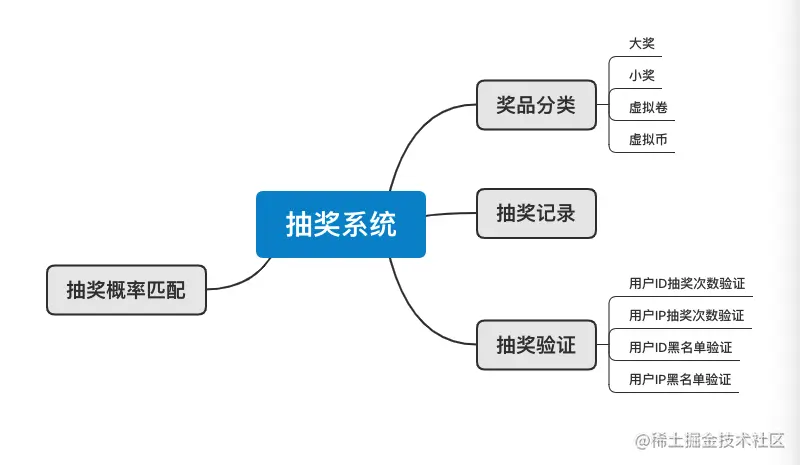3592

# 准备工作# 小试牛刀第一步

``````title: { type: DataTypes.STRING, comment: '奖品名称' },
prize_num: { type: DataTypes.INTEGER, comment: '奖品数量' }, //0 无奖品，>0限量，<0无限量
left_num: { type: DataTypes.INTEGER, comment: '剩余奖品数量' },
prize_code: { type: DataTypes.STRING, comment: '中奖的概率' }, //0~9999
prize_time: { type: DataTypes.INTEGER, comment: '发奖周期' }, //抽奖活动持续多少天
img: { type: DataTypes.STRING, comment: '奖品图片' },
displayOrder: { type: DataTypes.INTEGER, comment: '位置序号' }, //小的排在前面
gType: {
type: DataTypes.INTEGER,
comment: '奖品类型',
}, //3 虚拟币，2 虚拟卷，1 实物 小，0 实物 大
sys_status: {
type: DataTypes.INTEGER,
comment: '状态',
}, // 0 正常 1 删除
time_begin: { type: DataTypes.DATE, comment: '开始时间' },
time_end: { type: DataTypes.DATE, comment: '结束时间' },
``````

``````router.use(Token.checkToken)

//用户参与次数验证
router.use(usersignService.checkUser)

//ip参与次数验证
router.use(ipService.checkIp)

//ip黑名单
router.use(ipBlacklistService.checkIp)

//用户黑名单
router.use(blacklistService.checkUser)

//抽奖接口
router.get('/getPrize', luckyConstructor.prizeGet)

//礼品的颁发
router.use(couponService.setCoupon)

//中奖纪录
router.use(recodingsService.createData)
``````# 正式开始

## 抽奖奖品的设计

``````if (!data || data.length <= 0) {
return
}
const list = JSON.stringify(data) //序列化
await ctx.redis.set('allList', list)
``````

### 注意一个小细节

``````const houseData = [
// 中奖概率分成一百份，24小时每个时间段的机会
//100 / (3*24) = 28
//剩下28份分给不同的时间段
0,0,0,
1,1,1,
2,2,2,
3,3,3,
4,4,4,
5,5,5,
6,6,6,
7,7,7,
8,8,8,
9,9,9,
10,10,10,10,10,10,
11,11,11,11,11,
12,12,12,
13,13,13,
14,14,14,14,14,
15,15,15,15,15,15,
16,16,16,16,16,16,
17,17,17,
18,18,18,
19,19,19,19,19,19,
20,20,20,20,20,20,20,
21,21,21,21,21,21,21,
22,22,22,22,22,22,22,
23,23,23,
]
``````

`````` // 重置发奖计划
async resetPrizeData(data) {
const { prize_num, left_num, prize_time, time_begin } = data.dataValues
data.dataValues.prize_data = JSON.stringify(this.newHash)
if (prize_num < 0 || left_num < 0) {
return
}
if (prize_time <= 0) {
return
}
const num = Math.floor(prize_num / prize_time)

//每一天的发奖计划重制
let time = dayjs(time_begin)
if (num >= 1) {
for (let index = 1; index <= prize_time; index++) {
this.hash[time.format('YYYY-MM-DD')] = num
}
const remainder = prize_num % prize_time
if (remainder) {
for (let index = 0; index < remainder; index++) {
const ran = Math.floor(Math.random() * (1, prize_time)) + 1
let t = dayjs(time_begin)
this.hash[t.format('YYYY-MM-DD')] += 1
}
}
for (const it in this.hash) {
this.setTime(it)
}
}
data.dataValues.prize_data = JSON.stringify(this.newHash)
}
//一天24小时的发奖计划
setTime(it) {
while (this.hash[it]) {
const day = dayjs(it)
this.hash[it]--
const ran = Math.floor(Math.random() * (0, 99))
const d = day.hour(houseData[ran])
const item = d.format('YYYY-MM-DD HH:mm:ss')
if (!this.newHash[item]) {
this.newHash[item] = 0
}
this.newHash[item] += 1
}
}

``````

## 开始抽奖

``````const num = Math.floor(Math.random() * 10000) //生成抽奖编码
let prizeList = await prizeService.getData(ctx)
if (!prizeList) {
prizeList = await prizeService.setRData(ctx)
}
let it
for (let index = 0; index < prizeList.length; index++) {
if (
prizeList &&
prizeList[index] &&
prizeList[index].prize_code > num &&
!ctx.bool
) {
it = prizeList[index]
break
}
}
if (!it) {
throw new successExpection('没有中奖，谢谢参与')
}
ctx.it = it
await next()
``````

## 发奖

``````  const item = await ctx.redis.hget('Pool', ctx.it.id)
if (item <= 0) {
throw new successExpection('没有中奖，谢谢参与')
}
const data = await ctx.redis.hincrby('Pool', ctx.it.id, -1)
if (data < 0) {
throw new successExpection('没有中奖，谢谢参与')
}
const prize = await Prize.findOne({
where: {
gType: num,
},
})
await prize.decrement(['left_num'])
``````

## 最后

••••••••••••